# Cupcakes

In a bowl was some cupcakes. Janka ate one third and Danka ate one quarter of cupcakes.

a) How many of cookies ate together?
b) How many cookies remain in a bowl?

Write the results as a decimal number and in notepad also as a fraction.

a =  0.58
b =  0.42

### Step-by-step explanation:

$a=\frac{1}{3}+\frac{1}{4}=\frac{7}{12}=0.58$
$b=1-a=1-\left(\frac{1}{3}+\frac{1}{4}\right)=\frac{5}{12}=0.42$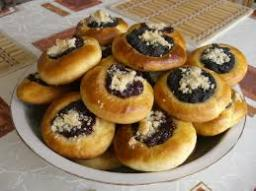Did you find an error or inaccuracy? Feel free to write us. Thank you!Tips to related online calculators
Need help to calculate sum, simplify or multiply fractions? Try our fraction calculator.

## Related math problems and questions:

• Janka and Danka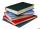Janka and Danka began to read the same day the books. Janka had 276 pages, Danka 204 pages. They agreed that both read every day the same number of pages until theirs books read. A) How many maximum pages they had to read the day to honor their agreement?
• CakesOn the bowl were a few cakes. Jane ate one-third of them, and Dana ate a quarter of those cakes that remained. a) What part (of the original number of cakes) Dana ate? b) At least how many cakes could be (initially) on the bowl?
• School dance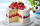Lucy and JoJo need to make 140 cupcakes for the school dance. Lucy made 3/5 of the cupcakes. JoJo made 42 cupcakes. What fraction of the cupcakes do Lucy and JoJo still need to make?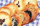In a cookie jar, 1/4 of the cookies are chocolate chip and 1/2 of the rest are peanut butter. What fraction of all the cookies are peanut butter?
• Pizza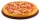Three siblings ordered one pizza. Miška ate a quarter of the whole pizza. Lenka ate a third of the rest and Patrik ate half of what Lenka had left. They had the rest packed up. How much of the pizza did they pack? Write the result as a fraction.
• Fraction to decimalWrite the fraction 3/22 as a decimal.
• Decimal to fractionWrite decimal number 8.638333333 as a fraction A/B in the basic form. Given decimal has infinite repeating figures.
• David numberJana and David train the addition of the decimal numbers so that each of them will write a single number and these two numbers then add up. The last example was 11.11. David's number had the same number of digits before the decimal point, the Jane's numbe
• Clothes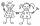Danka and Janka collect clothes. Danka had 9 more than Janka, so she gave her 7. Which now has more cloth and how many?
• Sisters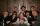Sisters Janka and Danka are saved together 220 CZK. On a trip to Janka wants to take a fifth of her savings and Danka's quarter. Then they will have 50 CZK on the trip. How much money saved Janka and Danka?
• Candies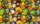There are 150 candies in the bowl. Ivan took 7 thirtieths, Charles 6 fifteenths, and Thomas 3 tenths. How many sweets are left in the bowl?
• Fraction and a decimalWrite as a fraction and a decimal. One and two plus three and five hundredths
• MichaelMichael had a bar of chocolate. He ate 1/2 of it and gave away 1/3. What fraction had he left?Mom bake cookies. Rolo took 2/9 of all cookies, Michal 3/9. How many cookies ate Rolo if Michal had 9.There are 12 pears in the bowl. Erik ate a third. How many pears remained in the bowl?Evaluate 2x+6y when x=- 4/5 and y=1/3. Write your answer as a fraction or mixed number in simplest form.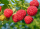Carrie picked 2/5 of the raspberries from the garden, and Robin picked some too.  When they were finished, 1/3 of the raspberries still needed to be picked.  What fraction of the raspberries did Robin pick? Use pictures, numbers or words and write your fi